### Second Law of Thermodynamics

For a closed system which is not in thermal equilibrium, the most probable consequence is that the entropy of the system is monotonically increasing until a state of thermal equilibrium has been reached . The traditional version of this theorem was given by THOMSON2.9 and PLANCK2.10: A thermo-mechanical circle process which performs only a conversion of heat from a heat capacitor to its equivalent work is impossible . Machines which violate this theorem are called perpetuum mobiles of second order. This can be expressed by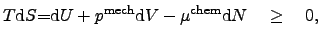(2.35)

where the equivalent of the increase of the disorder of the system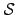is greater then the maximum of the performed work of the system.

Many thermodynamic problems in microelectronics deal among others with systems in which chemical reactions are often neglected. Hence, the number of particles in the system can be assumed to be constant. So, for a given systemwhere the number of particles remains constant (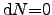), the second law of thermodynamics can be equivalently formulated as the so called thermodynamic identity (2.36) and its conversions (2.37):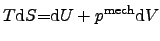(2.36)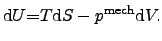(2.37)

Hence, the energy increase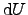of a system can be expressed by the sum of the heat transfer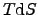to the system and the mechanical work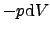done on the system.

Since every thermodynamic system can be assumed to be a dissipative system, the energy can be split into reversible and irreversible state variable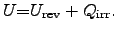(2.38)

For instance, if the dissipated heat is collected and again converted to energy, the conversion process has also a finite power loss, and the originally dissipated heat cannot be completely restored, otherwise a perpetuum mobile has been constructed which is impossible because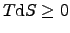has to be fulfilled. Introducing (2.38) to (2.36) and (2.37), the thermodynamical identities can be expressed by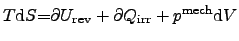(2.39)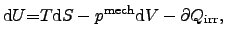(2.40)

where the newly introduced internal energy changeis reduced by the irreversible part which has been dissipated to the surrounding systems. For the reversible case, the latter equations become again (2.36) and (2.37).

Stefan Holzer 2007-11-19